# Plus Two Chemistry Chapter Wise Questions and Answers Chapter 8 The d and f Block Elements

Students can Download Chapter 8 The d and f Block Elements Questions and Answers, Plus Two Chemistry Chapter Wise Questions and Answers helps you to revise the complete Kerala State Syllabus and score more marks in your examinations.

## Kerala Plus Two Chemistry Chapter Wise Questions and Answers Chapter 8 The d and f Block Elements

### Plus Two Chemistry The d and f Block Elements One Mark Questions and Answers

Question 1.
The element of the 3d series which is not regarded as a transition element is ____________ .
Zinc

Question 2.
Say TRUE or FALSE:
Cr2+ compounds are strong oxidising agents
False

Question 3.
Which of the following has the least magnetic moment?
(a) Cu2+
(b) Ni2+
(c) Co2+
(d) Fe2+
(a) Cu2+Question 4.
The oxidation state of Cr in K2Cr2O7 is _________
+ 6

Question 5.
Lanthanoid contraction implies
(a) Decrease in density.
(b) Decrease in mass.

Question 6.
Which of the following species are paramagnetic?
(a) Fe2+
(b) Zn
(c) Hg2+
(d) Ti4+
(a) Fe2+

Question 7.
The approximate percentage of iron in misch metal is____
5

Question 8.
The maximum oxidation state shown by Mn in its compounds is _____
+ 7Question 9.
The acidic, basic or amphoteric nature of Mn2O7, V2O5 and CrO are respectively
acidic, amphoteric and basic

Question 10.
The correct order of oxidising power of the following is
(a) Cr2O72- > MnO4 > VO2+
(b) MnO4 > Cr2O72- > VO2+
(c) VO2+ >MnO4 > Cr2O72-
(d) MnO4 > VO2+ > Cr2O72-
(e) Cr2O72- > VO2+ > MnO4
(b) MnO4 > Cr2O72- > VO2+

### Plus Two Chemistry The d and f Block Elements Two Mark Questions and Answers

Question 1.
Transition metals can form complexes.

1. Is the statement true?
2. Justify.
3. Give two examples of complexes.

1. Yes
2. Transition elements form complex compounds due to the comparatively smaller size of the metal ion, their high ionic charges and the availability of d-orbital for the bond formation.
3. [Fe(CN)6]3-, [Cr(NH3)6]3+Question 2.
Consider the statement: Scandium is a transition element but zinc is not.

1. Do you agree with his statement?

1. Yes.

2. Scandium has incompletely filled 3d orbitals in its ground state (3d0), it is regarded as a transition element. On the other hand, zinc atom has completely filled d orbitals (3d10) in its ground state as well as in its oxidised state. Hence it is not regarded as a transition element.

Question 3.
Transition elements show variable oxidation state.

1. Give reason.
2. Illustrate with an example.

1. The variable oxidation states of transition elements is due to the participation of ns and (n-1) d electrons in bonding,
2. E.g.: The outer configuration of Mn is 3d5,4s2 it exhibits all the oxidation states from +2 to +7.

Question 4.
Cu+ is colourless while Cu2+ is coloured in aqueous solution.

1. Is it true?
2. What are the reasons for this?

1. Yes.
2. In Cu+ it is 3d10,4s0 and there is no d-d transition. Hence no colour. But in Cu2+ it is 3d9, 4s0 it has d-d transition and hence it has colour.Question 5.
The highest oxidation state of a metal is exhibited in its oxide or fluoride only.

1. Do you agree?
2. If yes, give reason.

1. Yes.
2. Due to very high electronegativity and small size oxygen and fluorine can oxidise the metal to its highest oxidation state.

Question 6.
What is misch metal? Give its use.
‘Misch metal’ is an alloy which consists of a lanthanoid metal (~ 95%) and iron (~ 5%) and traces of S, C, Ca and Al. It is used in Mg-based alloy to produce bullets, shell and lighter flint.

Question 7.
Some ions of transition metals are given below. Categorize them into those which are coloured or not. Justify your answer.
Ti3+, V2+, Sc3+, Mn2+, Ti4+, Cu2+, Zn2+, Cu+

 Coloured ions Colourless ions Ti3+ Sc3+ V2+ Ti4+ Mn2+ Zn2+ Ca2+ Cu+
• In Ti3+, V2+, Mn2+, Ca2+ d-d transition is possible. Hence they have colour.
• In Sc3+ and Ti4+(3d0), Zn2+ and Cu+(3d10) d-d transition is not possible.

Question 8.
Why are Mn2+ compounds more stable than Fe2+ toward oxidation to their +3 state?
The electronic configuration of Mn2+ is 3d5 , which is stable due to half-filled sub-shell. Hence, Mn2+ is not easily oxidised to Mn3+. On the other hand, Fe2+ has electronic configuration 3d6 . After losing one electron it changes to Fe3+ which has stable 3d5 electronic configuration. Hence, Fe2+ is relatively easily oxidised to Fe3+Question 9.
Explain briefly how +2 state becomes more and more stable in the first half of the first row transition elements with increasing atomic number?
Except Sc, the most common oxidation state of the first row transition elements is +2 which arises from the loss of two 4s electrons. The +2 state becomes more and more stable in the first half of first transition elements with increasing atomic number because 3d orbitals acquires only one electron in each of the five 3d orbitals.

Question 10.
In what way is the electronic configuration of the transition elements different from that of the nontransition elements?
Transition elements have partially filled d-dubshell belonging to penultimate energy level whereas non-transition elements do not have any partially filled d-subshell. In non-transition elements, the last electron enters the s or p-subshell whereas in transition elements the last electron enters the d-subshell of penultimate energy level.

Question 11.
Predict which of the following will be coloured aqueous solution?
Ti3+ , V3+ cu+, Sc3+ , Mn2+, Fe3+ and CO2+ and MnO4. Give reasons for each.
Any ion that has partially filled d-orbitals is coloured due to d-d transition in visible light. MnO4 has purpule colour due to charge-transfer. Cu+ (d10 ) and Sc3+ (d0) are white since there is no d-d transition.

Question 12.
Use Hund’s rule to derive the electronic configuration of Ce3+ ion and calculate its magnetic moment on the basis of ‘spin-only’ formula.
Ce (Z = 58) = 54[Xe]4f15d16s2
Ce3+ = 54[Xe]4f1
It has one unpaired electron.
µ = $$\sqrt{n(n+2)}$$ B.M.
µ = $$\sqrt{1(1+2)}=\sqrt{3}$$ B.M = 1.732 B.M.Question 13.
Among the following transition metal compounds which are coloured. Why?
TiO, TiO2, ZnSO4, MnCl2, CrCl3

• TiO → Coloured
• MnCl2 → Coloured
• CrCl3 → Coloured

Question 14.
In the series Sc (Z = 21) to Zn (Z = 30), the enthalpy of atomisation of zinc is the lowest. Why?
Zinc has weaker interparticle forces due to fully filled d-subshell (3d10).

Question 15.
Fill in the blanks: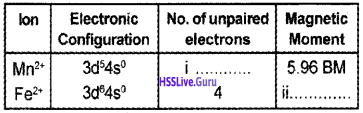i. 5

ii. 4.90 BM

### Plus Two Chemistry The d and f Block Elements Three Mark Questions and Answers

Question 1.
Explain why

1. Transition metals have higher density than alkaline earth metals?
2. Transition metals are less electropositive than alkaline earth metals?
3. Transition elements exhibit higher enthalpies of atomisation?

1. Transition metals have smaller atomic size and stronger interparticle attractive forces (metallic bond) than alkaline earth metals and hence have higher density than alkaline earth metals.

2. Transition metals due to their smaller atomic size and greater effective nuclear charge, have higher ionisation energy than alkaline earth metals and hence are less electropositive.

3. Transition elements have very strong interatomic bonds due to their small size and also due to the presence of large number of unpaired electrons in their atoms. As a result, they have very high enthalpies of atomisation.Question 2.
Two students wrote the electronic configuration of Chromium (Z = 24) as shown below:
Student A: [Ar] 4s2, 3d4
Student B : [Ar] 4s1 3d5

1. Pick out the correct electronic configuration and justify.
2. Calculate the spin only magnetic moments of Sc3+, Mn2+, Fe3+ and find out which is more paramagnetic.

1. [Ar] 3d54s1 . Due to the extra stability of the half-filled 3d level.

2. Sc3+ – n = 0 µ = 0 BM
Mn2+ – n = 5 µ = $$\sqrt{5(5+2)}=\sqrt{35}$$ = 5.92 BM
Fe3+ – n = 5 µ = $$\sqrt{5(5+2)}=\sqrt{35}$$ = 5.92 BM

### Plus Two Chemistry The d and f Block Elements Four Mark Questions and Answers

Question 1.
Some information regarding a particular compound is given below.
A deep purple black crystal which is moderately soluble in water at room temperature and it is prepared from pyrolusite.

1. Identify the compound.
2. Give the chemical reactions involved in the preparation of this compound.
3. Upon heating to 746 K, what happens to this compound? Give the equation.

1. KMnO4
2. 2MnO2 + 4KOH + O2 → 2K2MnO4 + 2H2O 3MnO42- + 4H+→ 2MnO4 + MnO2 + 2H2O
3. Potassium permanganate on strong heating gives potassium manganate, manganese dioxide and oxygen.
2KMnO4→ K2MnO4 + MnO2 + O2

Question 2.
Consider the statement: All transition elements form coloured compounds.

1. Do you agree?
2. Name three transition elements which cannot form coloured compounds.
3. What is the reason for colour of transition metal compounds?

1. No.
2. Zn, Cd and Hg.
3. The colour of transition compound is due to d-d transition. The colour observed corresponds to the complementary colour of the light absorbed.Question 3.
Complete the table: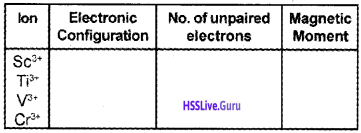Question 4.
The size of the atoms from Lanthanum to Lutetium, shows a steady and slow decrease in atomic size.

1. Do you agree with it?
2. What are the causes for this?
3. What are the consequences of this phenomenon?

1. Yes.

2. It is due to Lanthanoid contraction. In Lanthanoid series the 4f electrons are being added in the antipenultimate shell. The 4f electrons have very poor shielding effect because of diffused shape of 4f orbitals and the effective nuclear charge experienced by each 4f electrons increases. Hence there is a regular decrease in radii with increase in atomic number.

3. Consequences of lanthanoid contraction are:

• Difficulty in separation of lanthanoids due to similarity in chemical properties.
• Similarity in size of elements belonging to same group of second & third transition series.

Question 5.
Zn, Cd, Hg are pseudo transition elements.

1. What do you mean by pseudo transition elements?
2. Write the outer electronic configuration of transition elements.
3. Write any four properties of transition elements,

1. Pseudo transition elements have completely filled d orbitals and will not exhibit the characteristic properties of true transition elements.

2. The outer electronic configuration of transition elements is (n-1) d1 – 10 ns1 – 2.

3. Four properties of transition elements.

• They form coloured compounds.
• They exhibit variable oxidation state.
• They form complex.
• They are good catalysts.Question 6.
In a demonstration experiment, the teacher prepared the following solutions.
Solution 1: ZnSO4 in water
Solution 2: CuSO4 in water

1. Which of the above solutions is coloured?
2. Explain the Chemistry behind it.

1. Solution 2: CuSO4 in water is coloured.

2. In CuSO4, Cu is in +2 oxidation state. Cu2+ has 3d9 electronic configuration and it can undergo d-d transition and hence has colour(blue). In ZnSO4, zinc is in +2 oxidation state. Zn2+ has the 3d10 configuration. It has no d-d transition and hence it is colourless.

Question 7.

1. What is the effect of heat on KMnO4
2. Give any two examples to show the oxidising property of KMnO4.

1. On heating, KMnO4 decomposes at 513 K into potassium manganate and oxygen gas is evolved.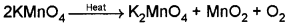2. Two examples to show the oxidising property of KMnO4

• In acid medium it oxidises iodide to iodine.
10I + 2MnO4 + 16H+ → 2Mn2+ + 8H2O + 5I2
• In neutral or faintly alkaline solutions it oxidises iodide to iodate.
2MnO4 + H2O + I → 2MnO2 + 2OH + IO3Question 8.
(a) Hf and Zr have similar ionic radii though they belongs to the 3rd and 4th transition series. Explain the reason for the anomalous behaviour.
(b) MnO4 & MnO42- are the ions of manganese. They differ in colour and magnetic nature.

1. How do this difference occured?

(a) This is a consequence of lanthanide contraction.

(b) Differ in colour and magnetic nature.
1. This is due to difference in number of electrons in d- subshell.
2.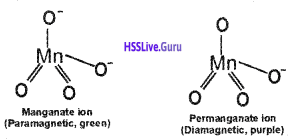Question 9.
Pyrolusite on heating with KOH in the presence of air gives a dark green compound (A). The solution of (A) on treatment with H2SO4 gives a purple coloured compound (B).
a) Identify ‘A’ and ‘B’ and write the balanced chemical equation.
b) Write two reactions to show the oxidising nature of ‘B’.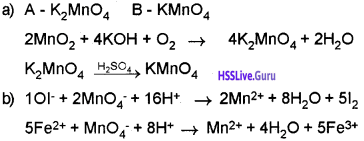Question 10.
Give the method of preparation and two examples for the oxidizing property of K2Cr2O7.
OR
Some d-block elements are given below:
Cr, Mn, Fe, Co, Ni
Identify the element which shows maximum paramagnetic behaviour.
K2 Cr2 O7 is prepared from chromate ore Fe Cr2 O4.
Step I: The powdered ore is heated with molten alkali to form soluble sodium chromate.

4 Fe Cr2 O4 + 16 NaOH + 7O2 → 8Na2CrO4 + 2Fe2O3 + 8H2O

Step II: Sodium chromate (Na2CrO4) is filtered and acidified with dil.H2SO4 to form sodium dichromate.

2Na2CrO4 + H2SO4 → Na2Cr2O7 + Na2SO4 + H2O

Step III: Na2Cr2O7 solution is treated with KCI to form

K2 Cr2 O7.
Na2Cr2O7 + 2 KCI —» K2 Cr2 O7 + 2NaCI

OR

Magnetic moment, µ = $$\sqrt{n(n+2)}$$ BM
Cr; n=6 µ = $$\sqrt{48}$$ = 6.93 BM
Mn; n=5 µ = $$\sqrt{35}$$ = 5.92 BM
Fe; n=4 µ = $$\sqrt{24}$$ = 4.90 BM
Co; n=3 µ = $$\sqrt{15}$$ = 3.87BM
Ni; n=2 µ = $$\sqrt{8}$$ = 2.83 BM
∴ Cr has maximum paramagnetic behaviour.

Question 11.

1. Even though usually transition elements exhibit +2 oxidation states, it changes from 0 to +8 in different compounds. What is the reason for it?
2. In the first transition series the atomic radius is decreasing from Sc to Cr and remains constant from Cr to Cu. From Cu to Zn it increases. Why?
3. The atomic radii of second and third transition series are same. Why?

1. The variable valency is due to the following reason. The energy difference between inner ‘d’ level and outers level is so small. Therefore electrons from ‘d’ level as well as ‘s’ level can take part in reaction.

2. Sc to Cr – Atomic radius decreases due to increase in nuclear charge. Cr to Cu – Due to a balance between the increased nuclear charge and increased screening effect atomic radii become almost constant.

Cu to Zn – Repulsive interactions between the paired electrons in d-orbitals become very dominant towards the end of the period and cause the expansion of electron cloud and thus result in increased atomic size.

3. Lanthanoid, Contraction.Question 12.
a) Calculate the magnetic moments of the following ions and predict its magnetic property.
Ti3+ (Z = 22)
Ni2+ (Z = 25)
Fe3+ (Z = 26)
Zn2+ (Z = 30)Question 13.
TiO, TiO2, ZnSO4, MnCl2, CrCl3
Arrange the above metal ions in the increasing order of their magnetic moment. (3)The increasing order of magnetic moment is TiO2 = ZnSO4 < TiO < CrCl3, MnCl2

Question 14.
How magnetic moment is calculated? How will you identify magnetism of an element from its electronic configuration?
By using the ‘spin-only’ formula, n = $$\sqrt{n(n+2)}$$ BM where ‘n’ is the number of unpaired electrons and ‘µ’ is the magnetic moment in Bohr Magneton (BM). If the element contains an unpaired electron it is paramagnetic. If all the electrons are paired and

### Plus Two Chemistry The d and f Block Elements NCERT Questions and Answers

Question 1.
Why are Mn2+ compounds more stable than Fe2+ toward oxidation to their +3 state?
The electronic configuration of Mn2+ is 3d5, which is stable due to half-filled sub-shell. Hence, Mn2+ is not easily oxidised to Mn3+. On the other hand, Fe2+ has electronic configuration 3d6. After losing one electron it changes to Fe3+ which has stable 3d5 electronic configuration. Hence, Fe2+ is relatively easily oxidised to Fe3+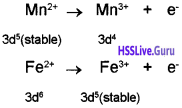Question 2.
Explain briefly how +2 state becomes more and more stable in the first half of the first row transition elements with increasing atomic number?
Except Sc, the most common oxidation state of the first row transition elements is +2 which arises from the loss of two 4s electrons. The +2 state becomes more and more stable in the first half of first row transition elements with increasing atomic number because 3d orbitals acquire only one electron in each of the five 3d orbitals (i.e., each d-orbital remains half-filled) and inter electronic repulsion is the least and nuclear charge increases.

In second half of first row transition elements, electron starts pairing up in 3d orbitals and hence there is inter electronic repulsion. (Ti2+ to Mn2+ electronic configuration changes from 3d2 to 3d5 but in second half i.e., Fe2+ to Zn2+ it changes from 3d6 to 3d10.)

Question 3.
In what way is the electronic configuration of the transition elements different from that of the non-transition elements?
Transition elements have partially filled d-subshell belonging to penultimate energy level whereas non-transition elements do not have any partially filled d- subshell. In non-transition elements, the last electron enters the s or p-subshell whereas in transition elements the last electron enters the d-subshell of penultimate energy level.

Question 4.
Predict which of the following will be coloured in aqueous solution?
Ti3+, V3+, Cu+, Sc3+, Mn2+, Fe3+ and CO2+ and MnO4-. Give reasons for each.
Any ion that has partially filled d-orbitals is coloured due to d-d transition in visible light. Ti3+ (d1), V3+ (d2) Mn2+ (d5), Co2+ (d8) are coloured. MnO4- has purpule colour due to charge-transfer. Cu+ (d10) and Sc3+ (d0) are white since there is no d-d transition.Question 5.
Use Hund’s rule to derive the electronic configuration of Ce3+ ion and calculate its magnetic moment on the basis of ‘spin-only’ formula.
µ = $$\sqrt{n(n+2)}$$ B.M.
μ = $$\sqrt{1(1+2)}$$ = $$\sqrt{3}$$ BM